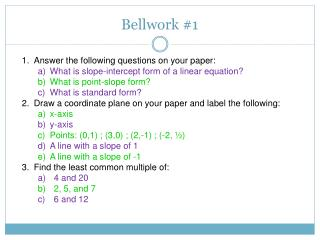Download PresentationBellwork #1

# Bellwork #1

Download Presentation## Bellwork #1

- - - - - - - - - - - - - - - - - - - - - - - - - - - E N D - - - - - - - - - - - - - - - - - - - - - - - - - - -
##### Presentation Transcript

1. Bellwork #1 • Answer the following questions on your paper: • What is slope-intercept form of a linear equation? • What is point-slope form? • What is standard form? • Draw a coordinate plane on your paper and label the following: • x-axis • y-axis • Points: (0,1) ; (3,0) ; (2,-1) ; (-2, ½) • A line with a slope of 1 • A line with a slope of -1 • Find the least common multiple of: • 4 and 20 • 2, 5, and 7 • 6 and 12

2. Bellwork #2 Solve each system of equations. y = x + 5 y = -x +7 y = 2x – 4 y = 4x – 10 y = 2x y = -x +15 Copy the diagram below onto your own paper. How many different lines can you draw connecting pairs of points in the diagram? • A • B • D • C

3. Unit 1 Tools of Geometry

4. Section 1.1 Points, lines, and planes

5. Vocabulary Challenge Can you guess which vocabulary word goes with which definition? Axiom Collinear points Coplanar Line Plane Point Postulate Space Write the above vocabulary words on your notes page.

6. A single location that has no size. • E • D Point • B • F • A • C A point is represented by a small dot and is named by a capital letter. A geometric figure is a set of points.

7. A series of points that extends in two opposite directions without end. Line t B A You can name a line by any two points on the line, such as: Read, “line AB” Another way to name a line is with a single lowercase letter, such as line t.

8. Points that lie on the same line. Collinear Points A B

9. Are points C,B, and A collinear? Yes Are points E, B, and D collinear? Yes Are points D, B, and A collinear? No What do you think: Are points C and E collinear?

10. A flat surface that has no thickness. Plane A plane contains many lines and extends without end in the directions of all its lines. You can name a plane by either a single capital letter or by at least three of its noncollinear points.

11. Name the plane represented by the front of the ice cube. plane AEF plane AEB plane ABFE

12. An accepted statement of fact. Postulate Or Axiom

13. What do you think: Are points C and E collinear?

14. Postulate 1-1 Through any two points there is exactly one line. No less! No more! t A B Line t is the only line that passes through points A and B.

15. What is one method to solve the following system of equations? 3x + 2y = 2 y = 1/2x - 3 We can solve by graphing. Why does graphing work to solve?

16. Postulate 1-2 If two lines intersect, then they intersect in exactly one point. At what point do these two lines intersect?

17. Postulate 1-3 If two planes intersect, then they intersect in exactly one line. What is the intersection of these two planes? DC

18. What is the intersection of plane HGFE and plane BCGF? Name two planes that intersect in plane BCFG and plane ABEF GF BF

19. Consider this three-legged music stand. A three-legged stand will always be stable. As long as the feet of the stand don’t lie in one line, the feet of the three legs will lie exactly in one plane.

20. Postulate 1-4 Through any three noncollinear points there is exactly one plane.

21. Copy this cube two times onto your own paper. (Be sure to label the vertices). 1. Shade the plane that contains A,B, and C. 2. Shade the plane that contains E, H, and C. 3. Name another point that is coplanar with points E, H, and C. Point B

22. Finding the least common multiple. x y z x2 y z x2y y z x xy xy2 xyz x2yz xy2 x2yz y xy xz2 z x3y z2 xy xy xy2 y2 xyz xy4 xyz2 xyz2 xy2 xy4z